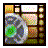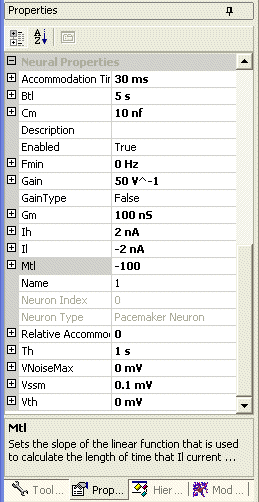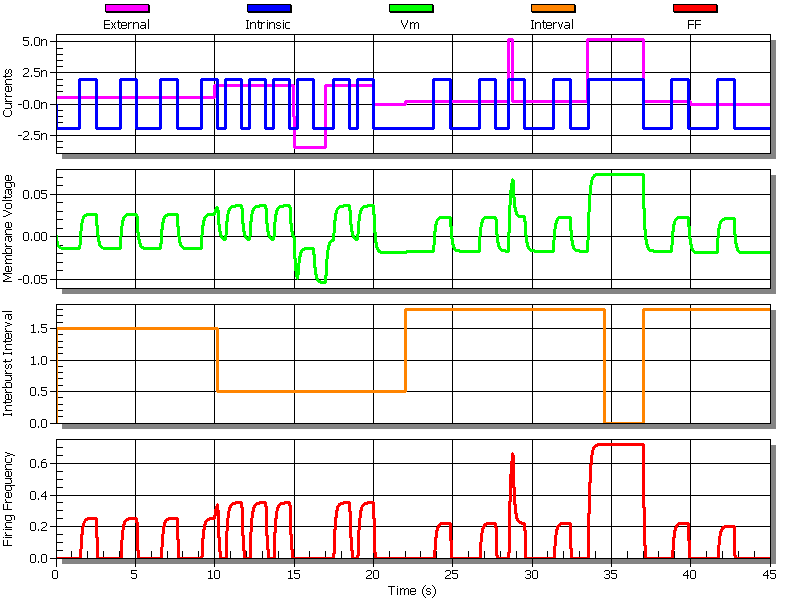# Pacemaker Neuron## Watch the video tutorial on this subject!

### Pacemaker Properties

Research into the details of locomotion and the rhythmic properties of some neural systems has shown that there is usually a subsystem that is able to produce rhythmic bursting to drive those behaviors. For example, the motion to move a leg would be controlled by one of these pacemaker units. Another good example would be in the heart, where the pacemaker would control the contraction of the heart muscle to pump blood. The pacemaker is usually a group of neurons that interact in a really complex way to produce this bursting property. However, this behavior can be modeled by a single neuron. Kandel described five properties for pacemakers that have been discovered through research. These properties are:

• When the pacemaker is sufficiently hyperpolarized, it is silent.
• When the pacemaker is sufficiently depolarized, it fires continuously
• Between these two extremes, it rhythmically produces a series of relatively fixed-duration bursts, and the length of the interval between bursts is a continuous function of the injected current.
• A transient depolarization which causes the cell to fire between bursts can reset the bursting rhythm.
• A transient hyper-polarization which prematurely terminates a burst can also reset the bursting rhythm.

These findings were used to come up with a set of rules that could produce that behavior in a neuron using two intrinsic currents (Beer, 1990). Ih is the high, depolarizing current and would tend to pull the membrane potential above threshold. Il is the low, hyperpolarizing current and would pull the membrane potential below threshold. The rules that use these two currents to produce the pacemaker behavior are:

• Ih is triggered whenever the cell goes above threshold or Il terminates, and it then remains active for a fixed length of time.
• Il is triggered whenever Ih terminates, and it then remains active for a variable amount of time whose duration is a function of the steady state membrane potential.
• Il is triggered whenever the membrane potential goes below a given lower threshold, and stays on until the threshold is exceeded.
• Only one of the intrinsic currents is active at any given time.Figure 1. Pacemaker Neuron Properties

The pacemaker neuron has all of the properties associated with a regular neuron: Cm, Gm, Vth, Fmin, and Gain. For a description of them please see the text that discusses the normal neuron. The properties that are unique for the pacemaker are listed below with a description of each.

Btl
The slope of the linear function that is used to calculate the length of time that Il current remains active.
Default value: 5 s.
Acceptable range:Greater than 0.

Ih
The depolarizing current that raises the membrane potential and causes the neuron to fire.
Default value: 2na.
Acceptable range:Any Value.

Il
The hyperpolarizing current that brings the membrane potential back down after it has been firing.
Default value: -2na.
Acceptable range:Any Value.

Mtl
The slope of the linear function that is used to calculate the length of time that Il current remains active.
Default value: -100.
Acceptable range:Any Value.

Th
This is the length of time that the Ih current remains active.
Default value: 1 S.
Acceptable range:Greater than 0.

Vssm
A lower steady state threshold. If the steady state voltage of the neuron goes below this value then the Il current is locked on until that voltage rises above this threshold.
Default value: 0mv.
Acceptable range:Any Value.

The steady state voltages mentioned above are calculated using Vss = (Iinput / Gn(1). Iinput is the input and external currents only. It does not include the intrinsic currents themselves. Also, The length of time that the Il current remains active is determined by this equation. Tl = (Mtl * Vss) + Btl  (2). So increasing Btl will increase the amount of time between pulses irregardless of the steady state voltage. Mtl determines in what way, and how much, the steady state voltage affects the length of time between pulses. What is desired is that by increasing the input current it will increase the pulse frequency. So if Vss is positive then Mtl should be negative in order to decrease the time that Il is active, and thus the length between pulses. Also, if Vss is negative then it means that the pacemaker neuron is being inhibited. If it is being inhibited strongly enough that Vss < Vssm then the pacemaker should be kept from firing at all. However, if enough depolarizing current is input into the pacemaker then the Il intrinsic current will no longer be strong enough to be able to pull the membrane voltage down enough to disable firing. This will mean that the neuron will fire continuously at that point until the input current is lessened.

### Neuron OutputFigure 2. Neural output that demonstrates the basic properties of the pacemaker neuron. The pacemaker neuron has the following properties: Btl = 2 s, Cm = 10 nf, Fmin = 0 Hz, Gain = 15 V^-1, Gm = 100 nS, Ih = 2 na, Il = -2 na, Mtl = -100 , Th = 1 s, Vssm = 0.1 mv , Vth = 0 mv The neuron was stimulated at the following times with the specified currents: 0-10 s with 0.5 na, 10-20 s with 1.5 na, 15-17 s with -5 na, 22-40 s with 0.2 na , 28.5-28.8 s with 5 na, 33.5-33.7 s with 5 na

Figure 2 demonstrates all the properties of a pacemaker neuron that were listed above. We will go through each one.

### Changing The Bursting Frequency

First notice that the initial 0.5 na stimulation causes the pacemaker to fire at a slow freqency. When the current is increased at 10 seconds the burst frequency of the neuron increases visibly. This demonstrates point 3 above. The firing frequency is a function of the current entering the cell. Lets look at the equations to try and understand how the interburst interval is calculated. We have Gm = 100 nS and an input current of 0.5 na. This gives us a steady state voltage of Vss = (0.5 na / 100 nS) = 5 mV. Plugging this into equation 2 gives Tl = (-100 * 5 mV) + 2 s = 1.5 s. Looking at the graph, two bursts occur within the first five seconds. Each burst lasts one second for a total of two seconds in burst mode. This leaves a total of three seconds between bursts. Divide 3 in half and you end up with 1.5 seconds between bursts. Also, The calculated interburst interval is displayed on the graph so you can see how it changes for different input current levels.

At 10 seconds we increase the input current to 1.5 na. This increases the steady state voltage to Vss = (1.5 na / 100 nS) = 15 mV. This gives us an interburst interval of Tl = (-100 * 15 mV) + 2 s = 0.5 s. You can see that the calculated interval drops to 0.5 at 10 seconds.

### Resetting The Bursting Frequency

Bursting is reset in three places in figure 2. The first occurs when a hyper-polarizing pulse is injected at 15 s. This pulls the membrane voltage below Vssm. We still get an intrinsic current oscillation, but it is no longer large enough to move the membrane voltage above its threshold and we do not get any firing while this hyper-polarizing current is being injected. Once this current is removed a new firing pattern with the same frequency emerges. The second place where the bursting is reset is when a depolarizing current is injected at 28.5 ms. A burst just finished when this new current was injected and this caused another burst to be fired immediately and reset the pattern. The third time was after the bout of continuous firing. You can also see that the interburst interval drops to zero during this period. When a pacemaker neuron is injected with a depolarizing current between bursts, it causes a new burst and resets the timing. But when a pacemaker is injected with a hyperpolarizing current during a pulse it terminates the pulse and resets the timing.

### Pacemaker Overview

The pacemaker neuron is the most complex neuron that is contained within this neural plug-in module. It has a variety of complex behaviors and this model only represents these properties in a very abstract manner. But this neuron, and all of it's features, play a key role in allowing simulated organisms be able to move their limbs in a synchronized manner.